Скачать презентацию Lecture 7 More Gravity and GPS Processing

61c497fdafd756929e7e605e58e257d0.ppt

• Количество слайдов: 42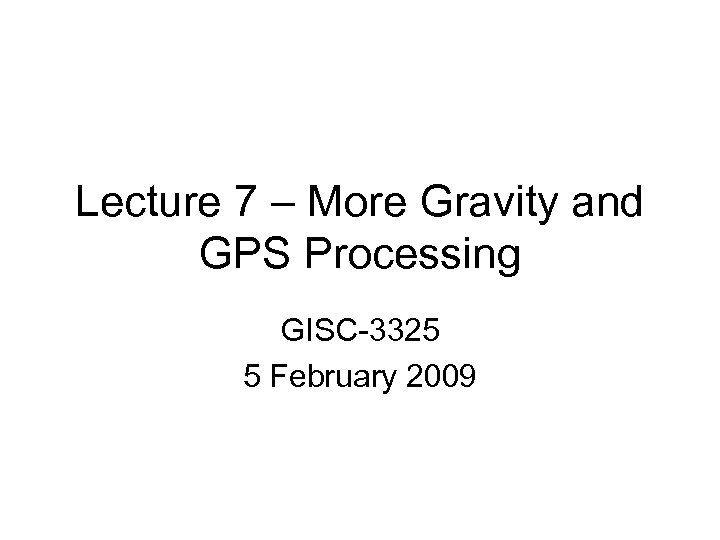Lecture 7 – More Gravity and GPS Processing GISC-3325 5 February 2009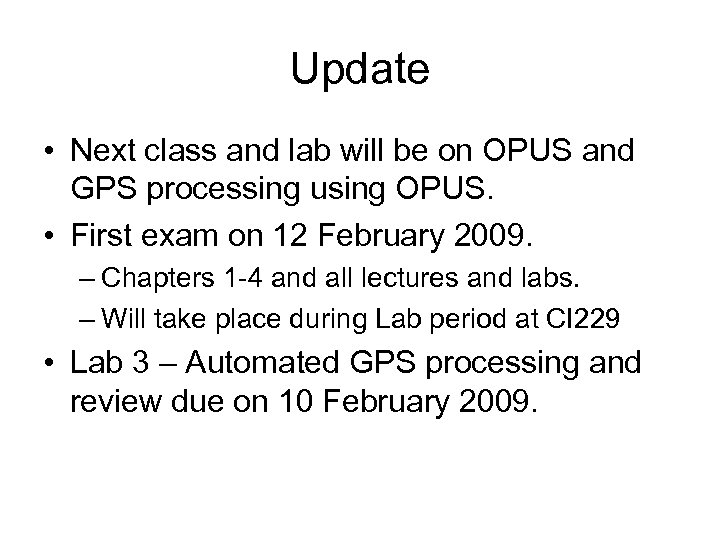Update • Next class and lab will be on OPUS and GPS processing using OPUS. • First exam on 12 February 2009. – Chapters 1 -4 and all lectures and labs. – Will take place during Lab period at CI 229 • Lab 3 – Automated GPS processing and review due on 10 February 2009.Reminder • You are responsible for the material listed as REQUIRED ADDITIONAL READING in the Class 5 section of the web page.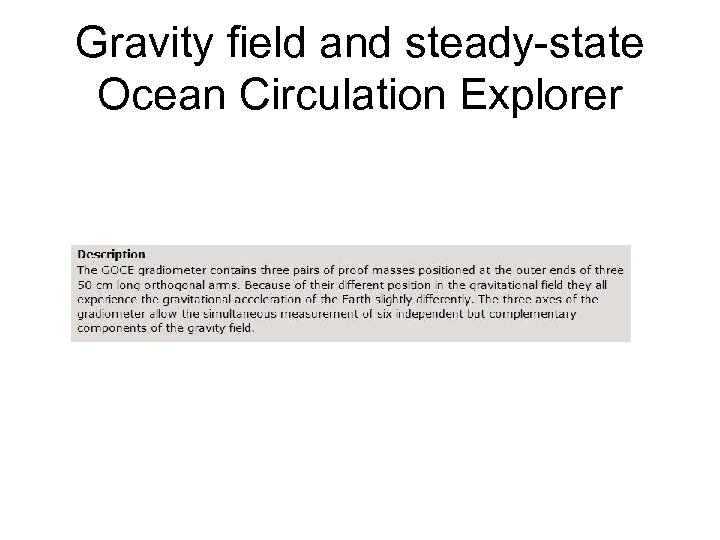Gravity field and steady-state Ocean Circulation Explorer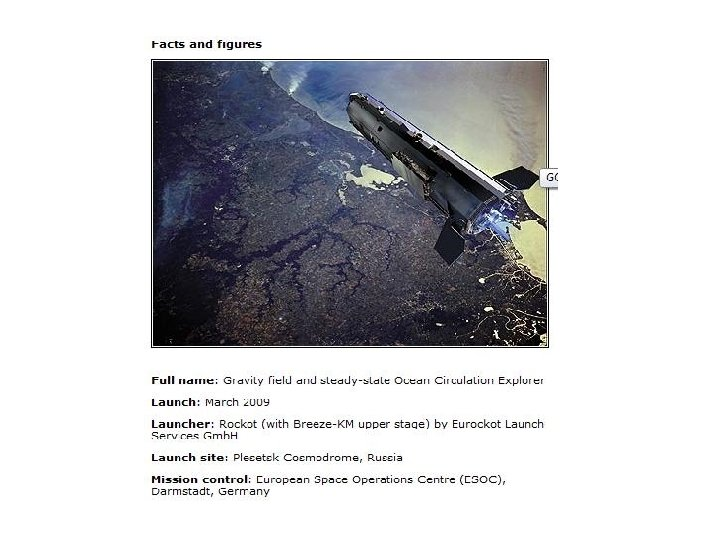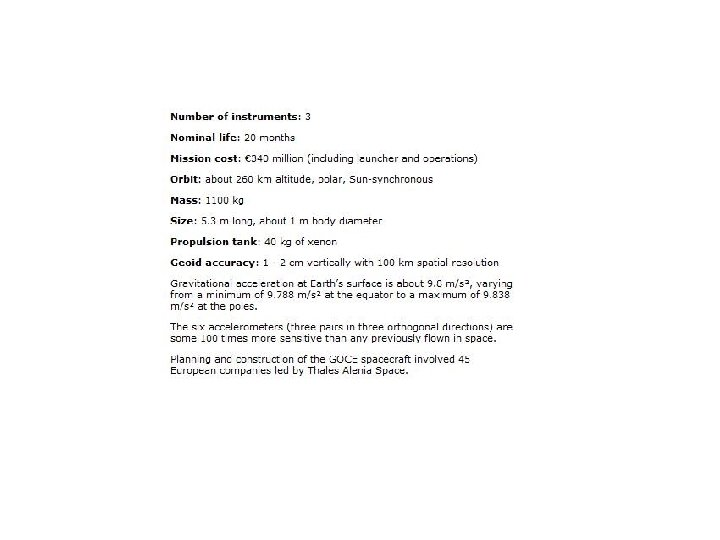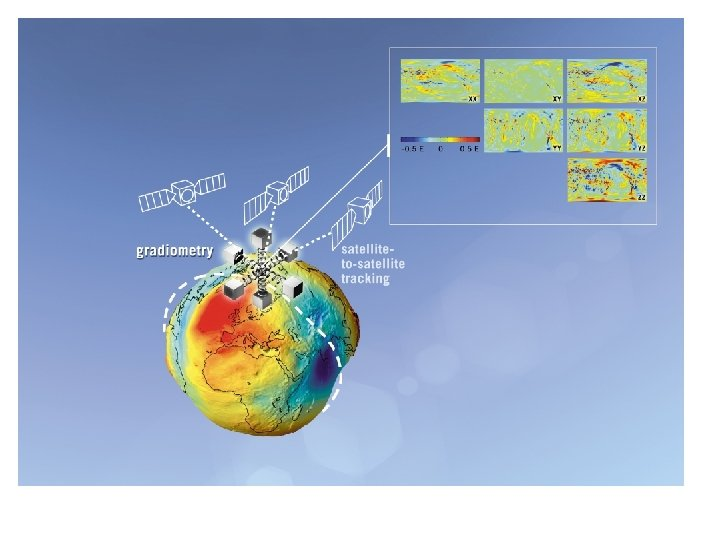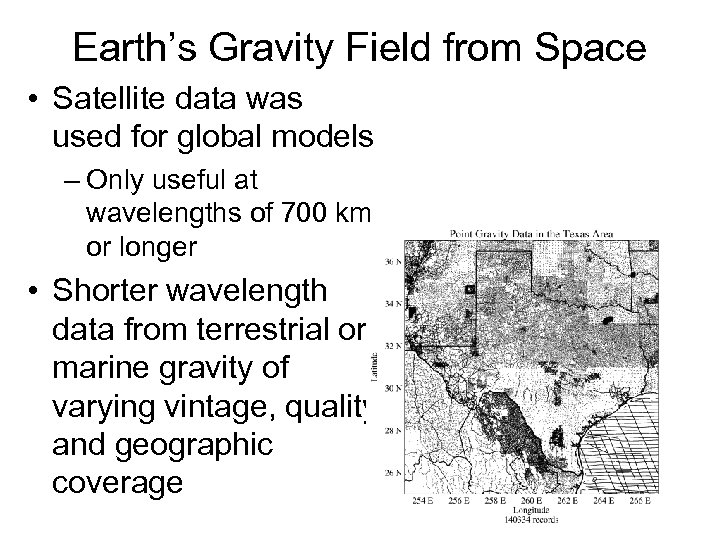Earth’s Gravity Field from Space • Satellite data was used for global models – Only useful at wavelengths of 700 km or longer • Shorter wavelength data from terrestrial or marine gravity of varying vintage, quality and geographic coverage Terrestrial and marine gravity data in NGS data base.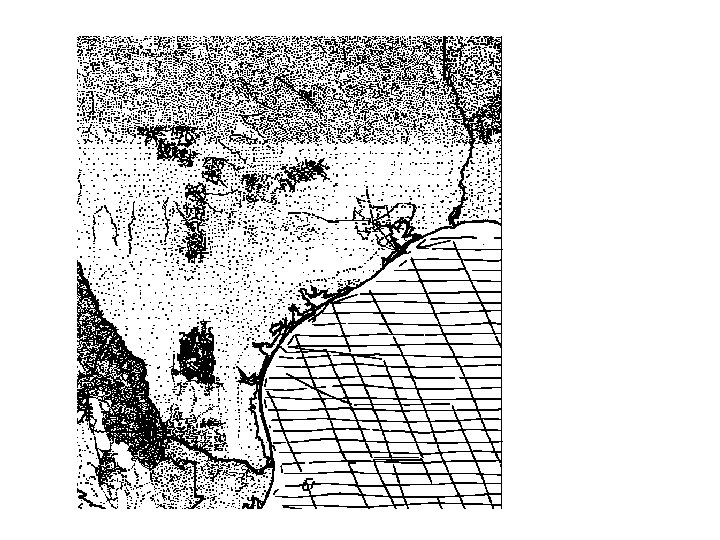Note the discontinuity at the shoreline.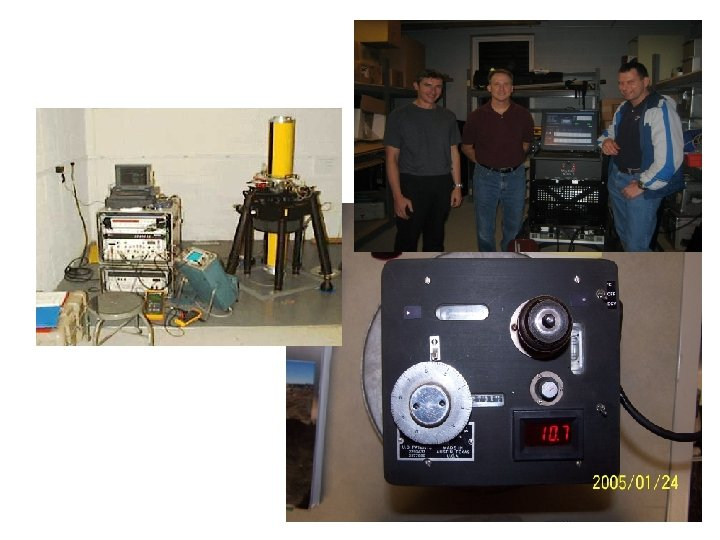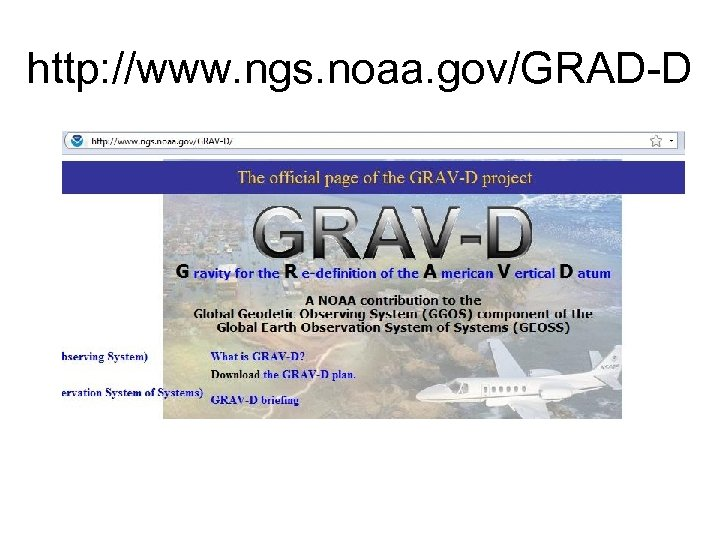http: //www. ngs. noaa. gov/GRAD-D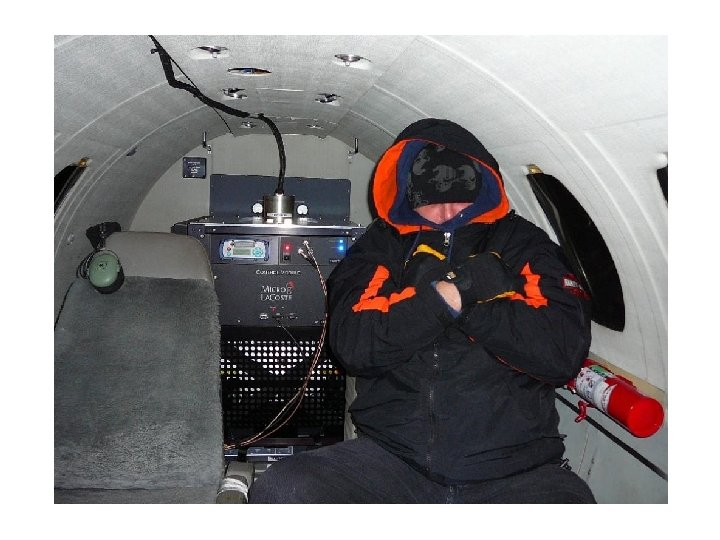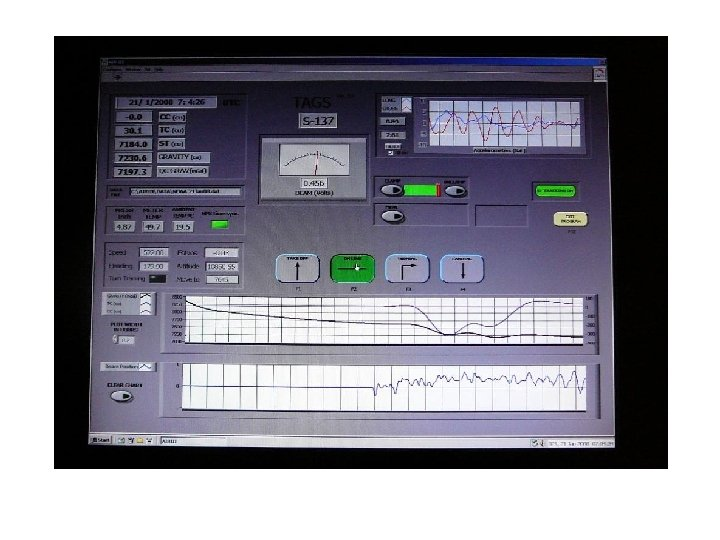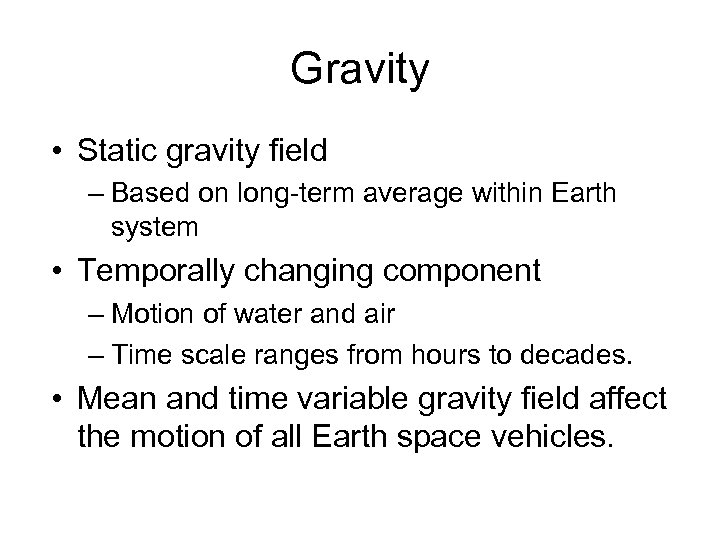Gravity • Static gravity field – Based on long-term average within Earth system • Temporally changing component – Motion of water and air – Time scale ranges from hours to decades. • Mean and time variable gravity field affect the motion of all Earth space vehicles.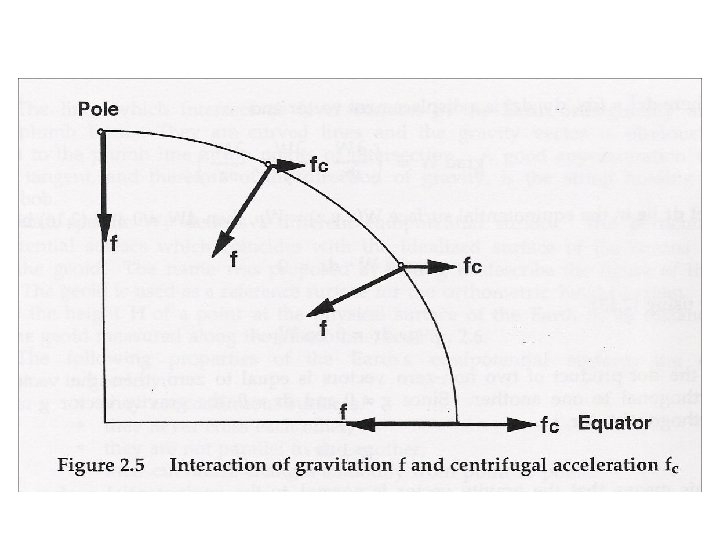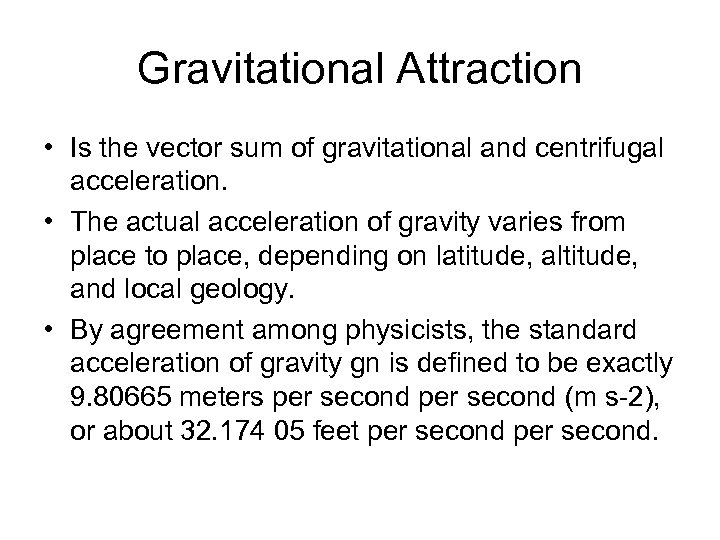Gravitational Attraction • Is the vector sum of gravitational and centrifugal acceleration. • The actual acceleration of gravity varies from place to place, depending on latitude, altitude, and local geology. • By agreement among physicists, the standard acceleration of gravity gn is defined to be exactly 9. 80665 meters per second (m s-2), or about 32. 174 05 feet per second.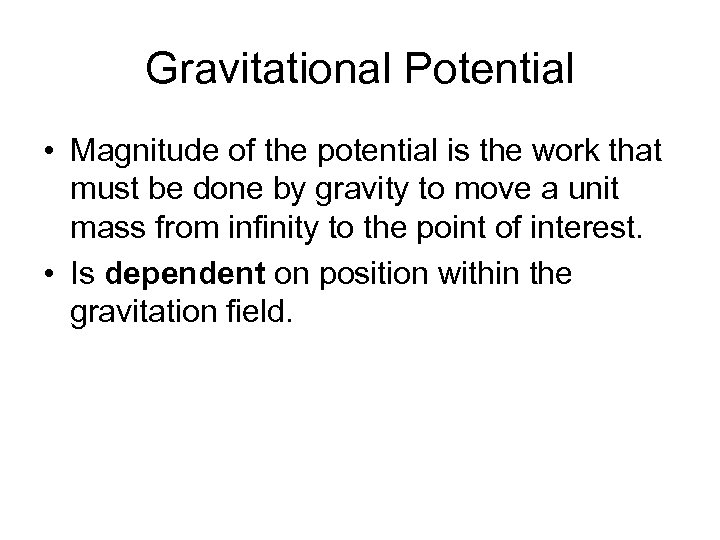Gravitational Potential • Magnitude of the potential is the work that must be done by gravity to move a unit mass from infinity to the point of interest. • Is dependent on position within the gravitation field.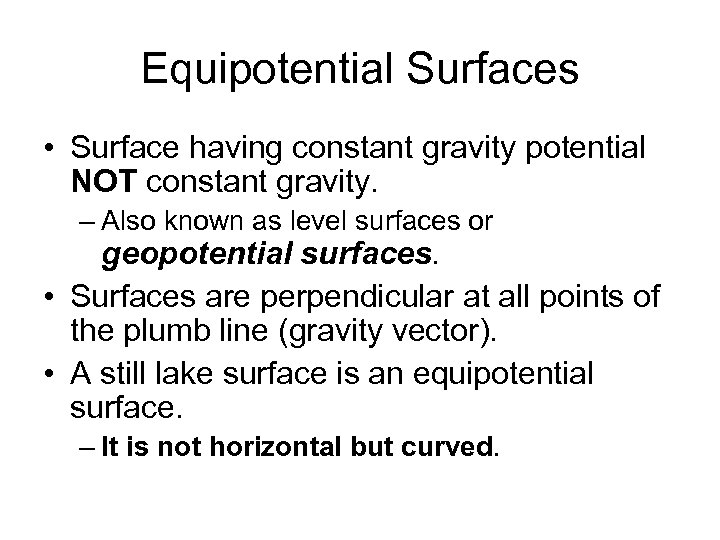Equipotential Surfaces • Surface having constant gravity potential NOT constant gravity. – Also known as level surfaces or geopotential surfaces. • Surfaces are perpendicular at all points of the plumb line (gravity vector). • A still lake surface is an equipotential surface. – It is not horizontal but curved.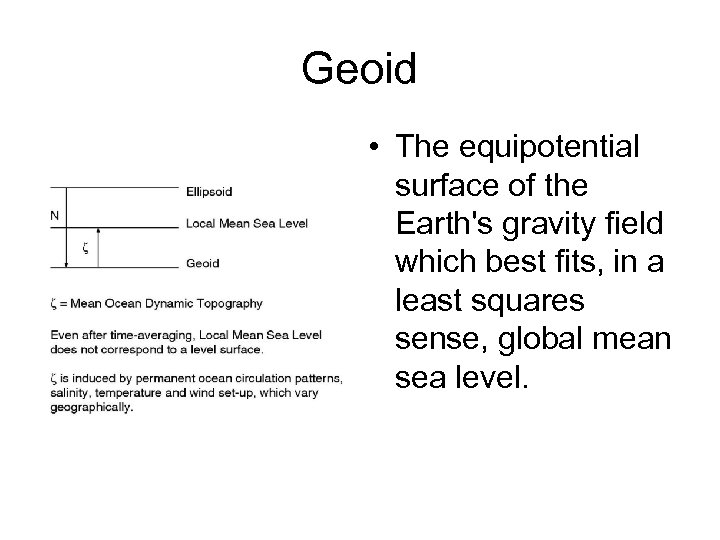Geoid • The equipotential surface of the Earth's gravity field which best fits, in a least squares sense, global mean sea level.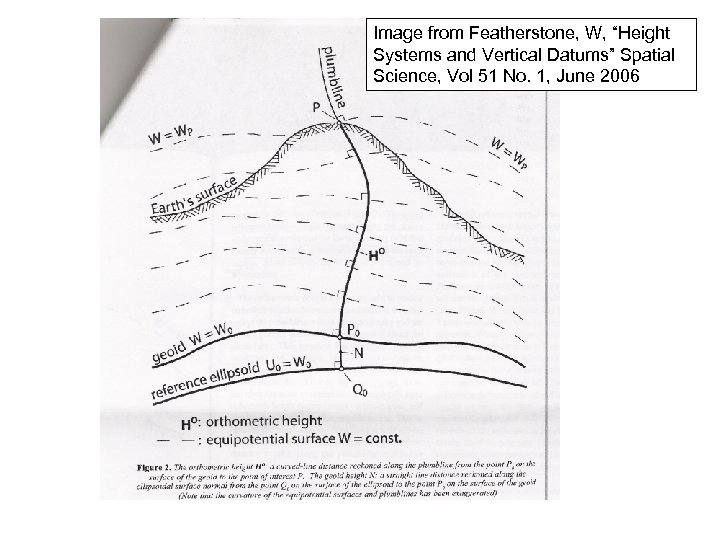Image from Featherstone, W, “Height Systems and Vertical Datums” Spatial Science, Vol 51 No. 1, June 2006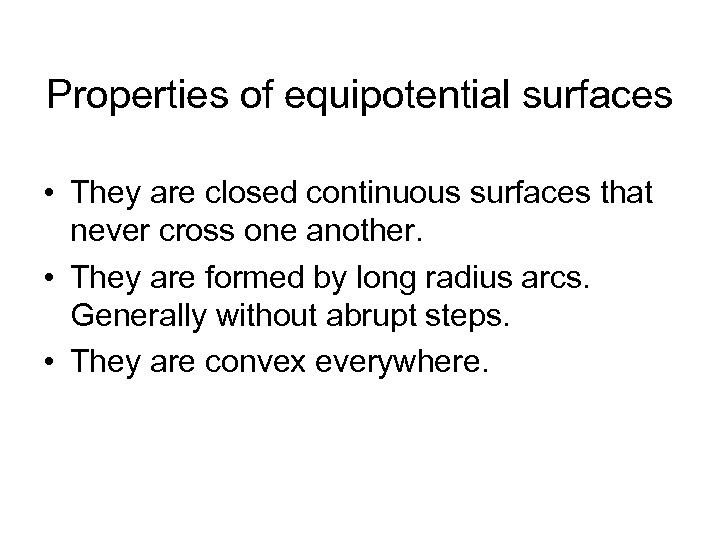Properties of equipotential surfaces • They are closed continuous surfaces that never cross one another. • They are formed by long radius arcs. Generally without abrupt steps. • They are convex everywhere.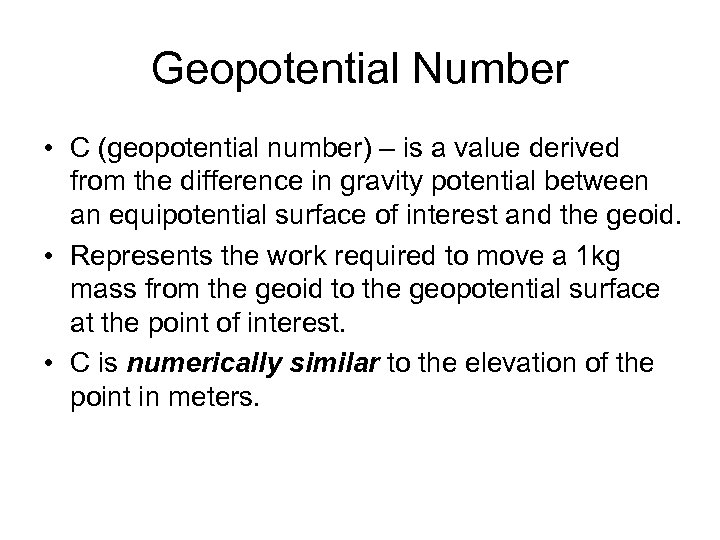Geopotential Number • C (geopotential number) – is a value derived from the difference in gravity potential between an equipotential surface of interest and the geoid. • Represents the work required to move a 1 kg mass from the geoid to the geopotential surface at the point of interest. • C is numerically similar to the elevation of the point in meters.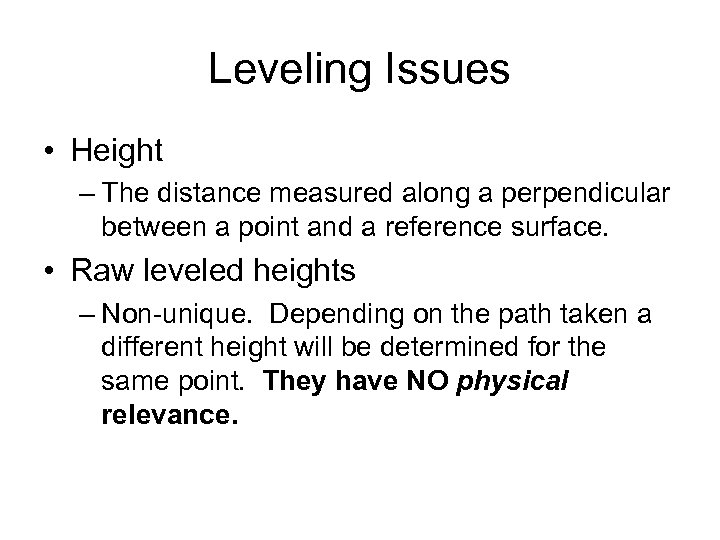Leveling Issues • Height – The distance measured along a perpendicular between a point and a reference surface. • Raw leveled heights – Non-unique. Depending on the path taken a different height will be determined for the same point. They have NO physical relevance.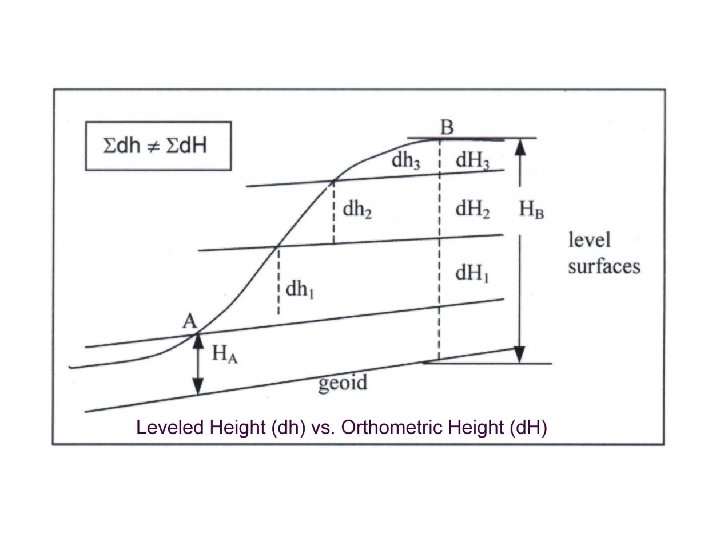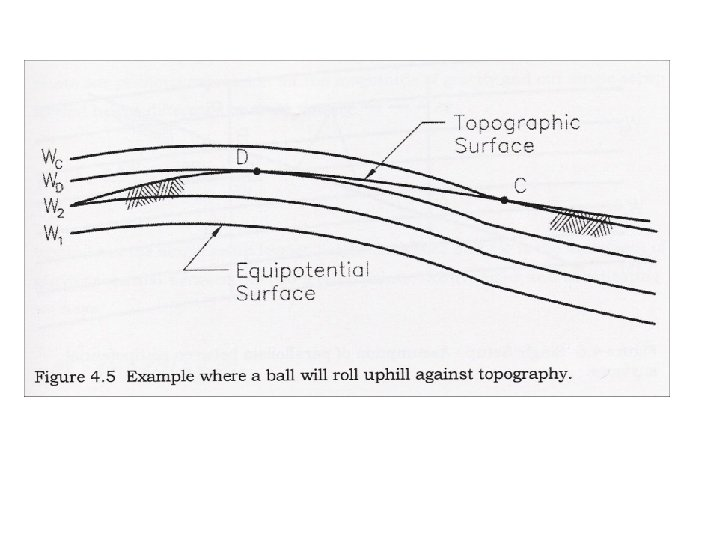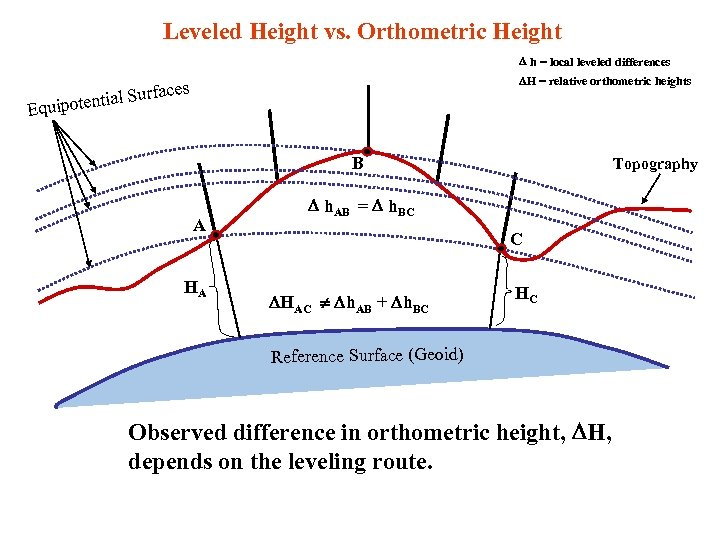Leveled Height vs. Orthometric Height h = local leveled differences H = relative orthometric heights faces ential Sur Equipot B A HA Topography h. AB = h. BC C HAC h. AB + h. BC HC Reference Surface (Geoid) Observed difference in orthometric height, H, depends on the leveling route.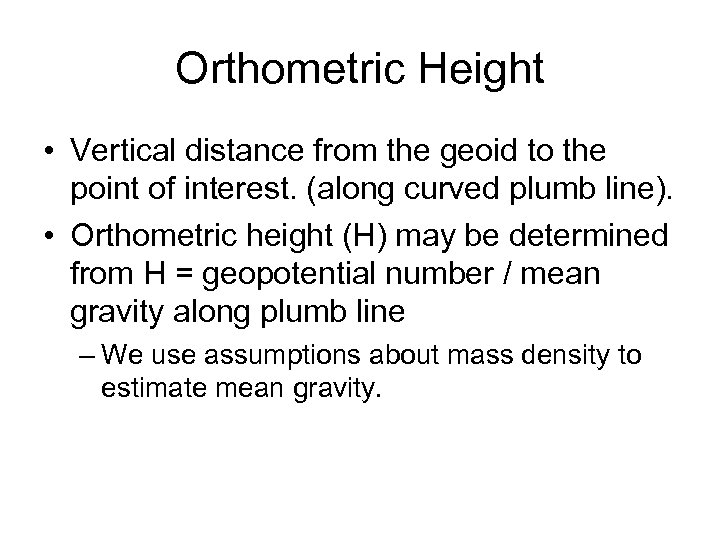Orthometric Height • Vertical distance from the geoid to the point of interest. (along curved plumb line). • Orthometric height (H) may be determined from H = geopotential number / mean gravity along plumb line – We use assumptions about mass density to estimate mean gravity.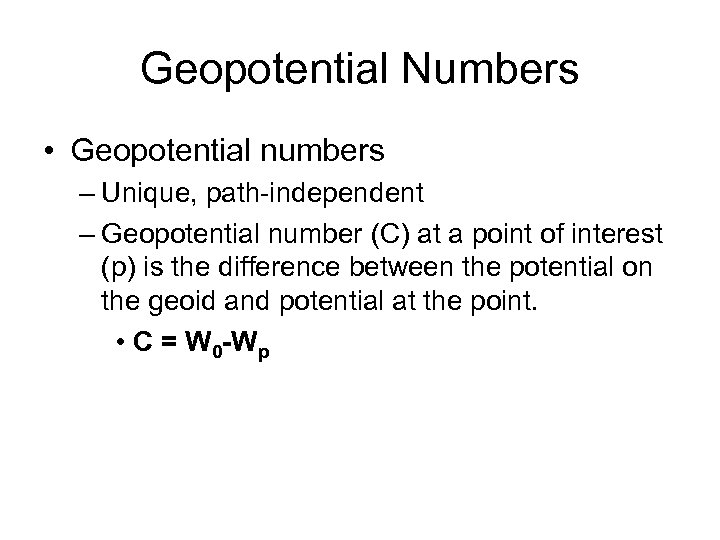Geopotential Numbers • Geopotential numbers – Unique, path-independent – Geopotential number (C) at a point of interest (p) is the difference between the potential on the geoid and potential at the point. • C = W 0 -Wp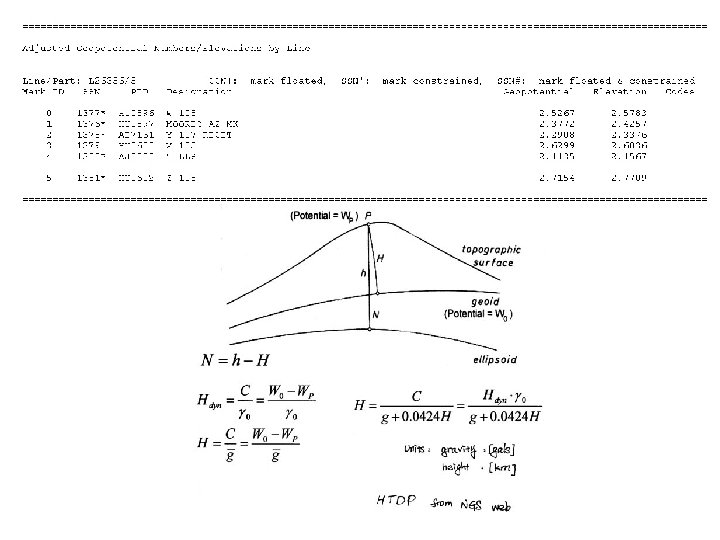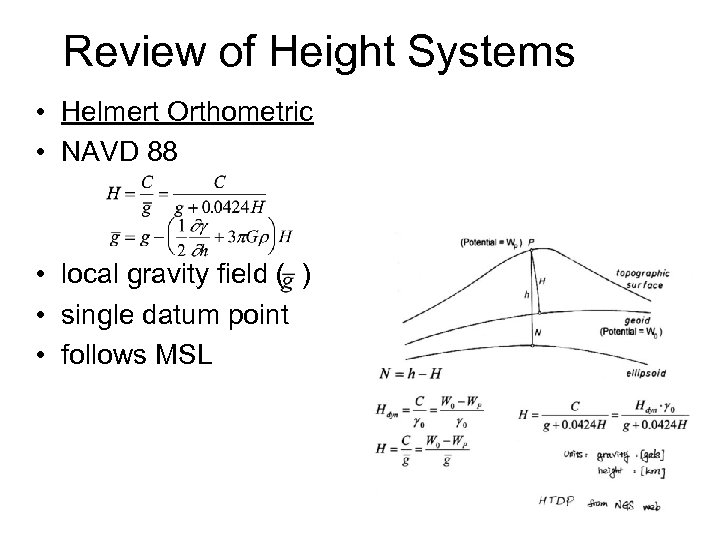Review of Height Systems • Helmert Orthometric • NAVD 88 • local gravity field ( ) • single datum point • follows MSL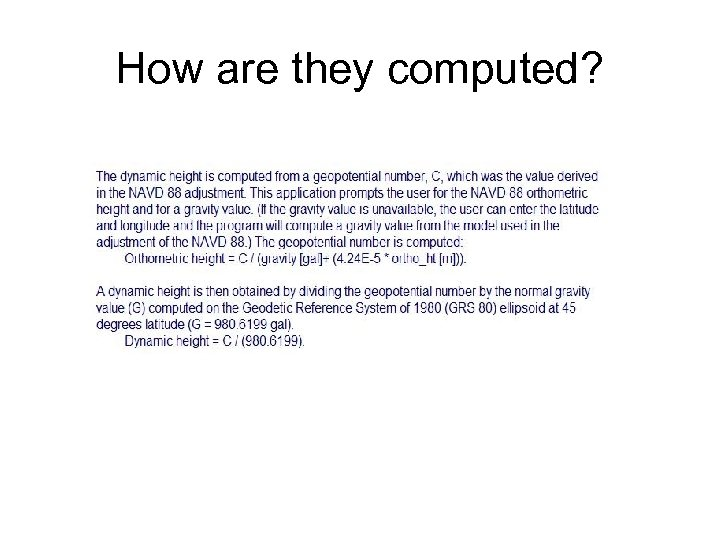How are they computed?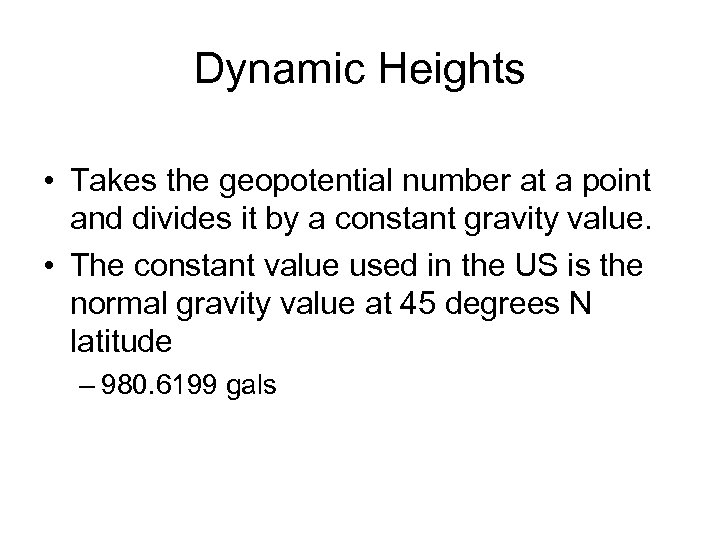Dynamic Heights • Takes the geopotential number at a point and divides it by a constant gravity value. • The constant value used in the US is the normal gravity value at 45 degrees N latitude – 980. 6199 gals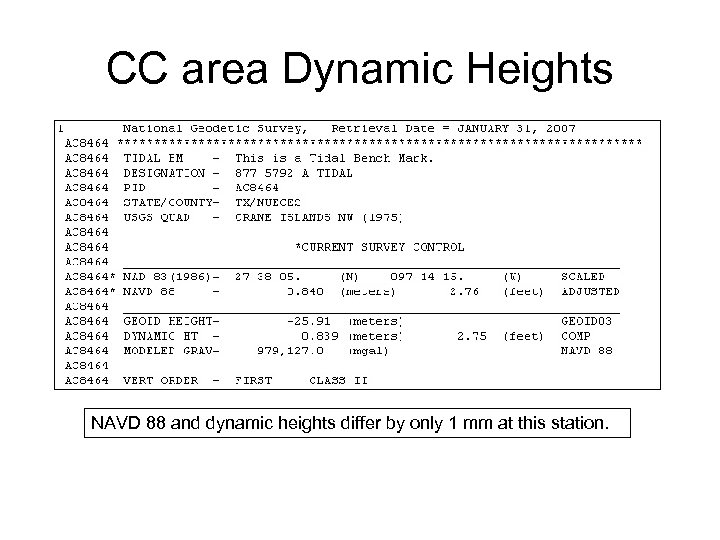CC area Dynamic Heights NAVD 88 and dynamic heights differ by only 1 mm at this station.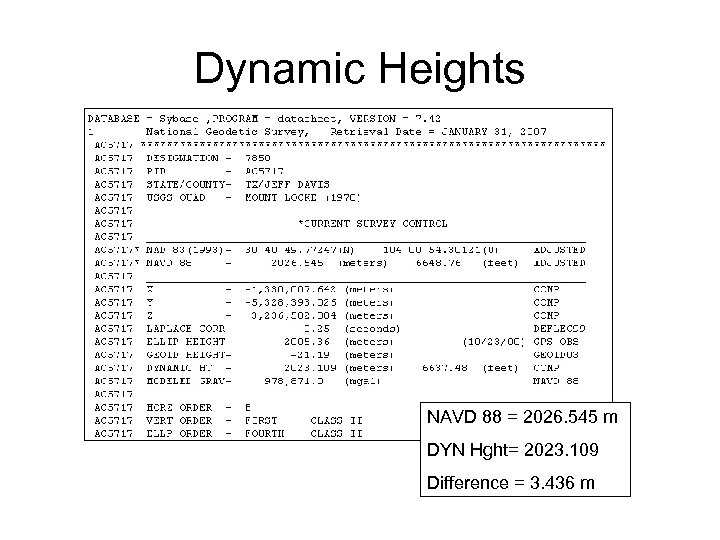Dynamic Heights NAVD 88 = 2026. 545 m DYN Hght= 2023. 109 Difference = 3. 436 m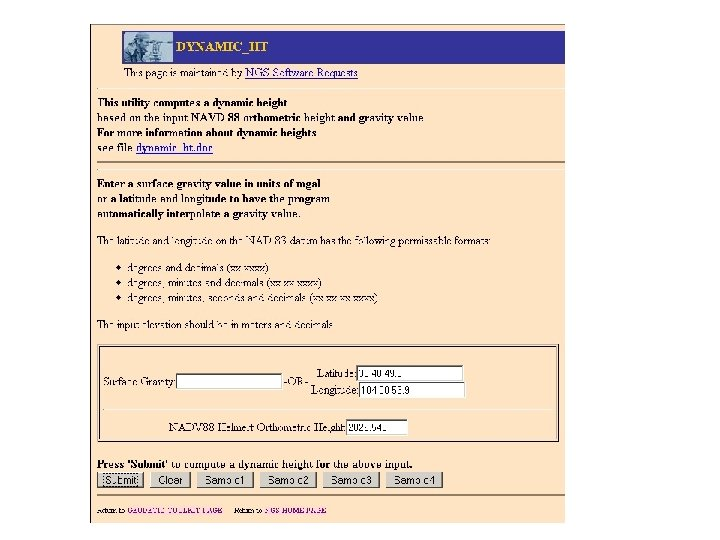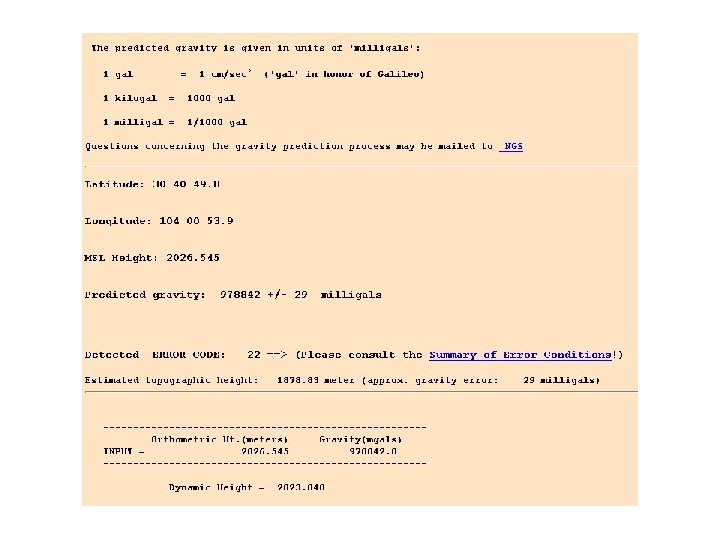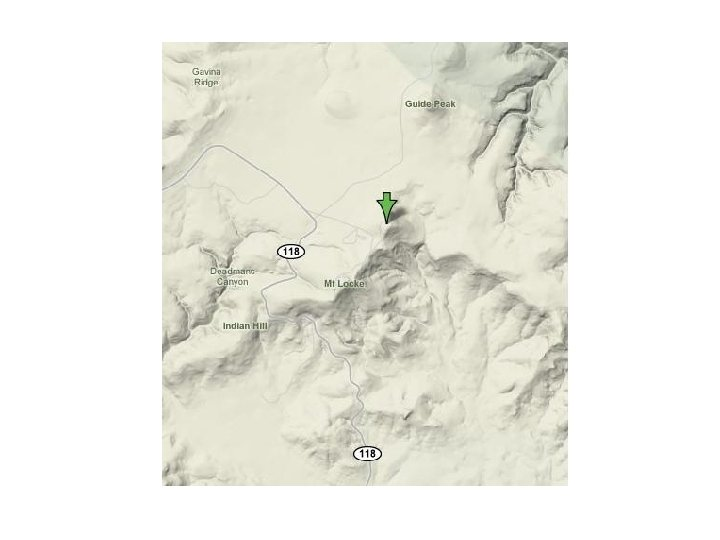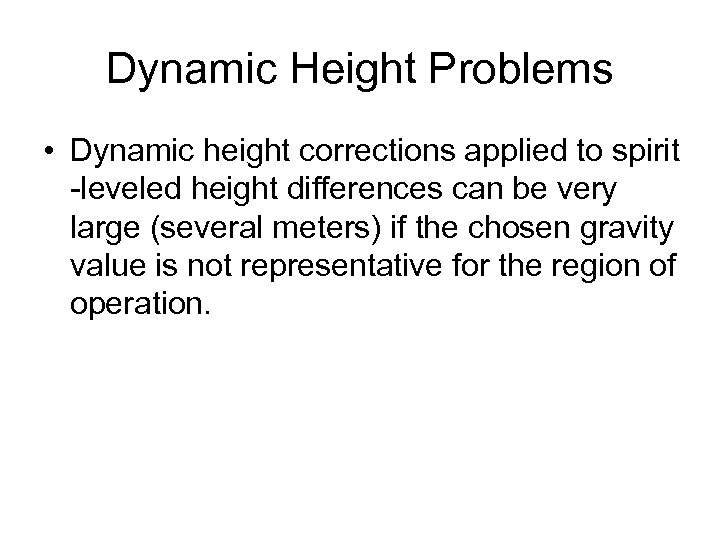Dynamic Height Problems • Dynamic height corrections applied to spirit -leveled height differences can be very large (several meters) if the chosen gravity value is not representative for the region of operation.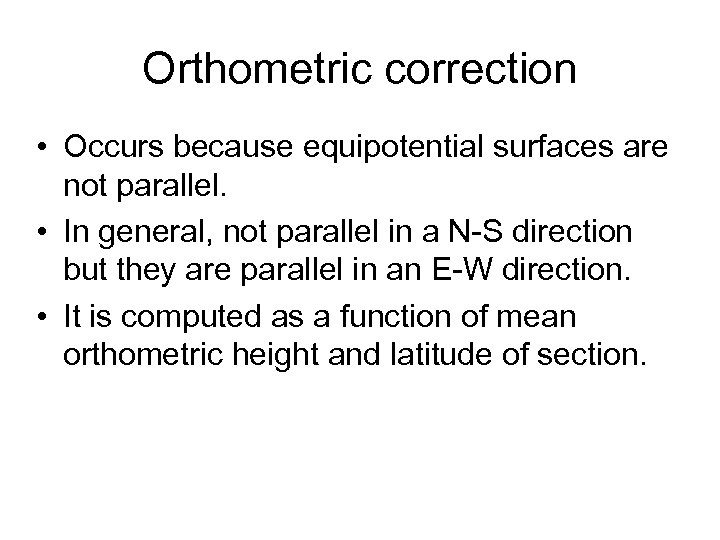Orthometric correction • Occurs because equipotential surfaces are not parallel. • In general, not parallel in a N-S direction but they are parallel in an E-W direction. • It is computed as a function of mean orthometric height and latitude of section.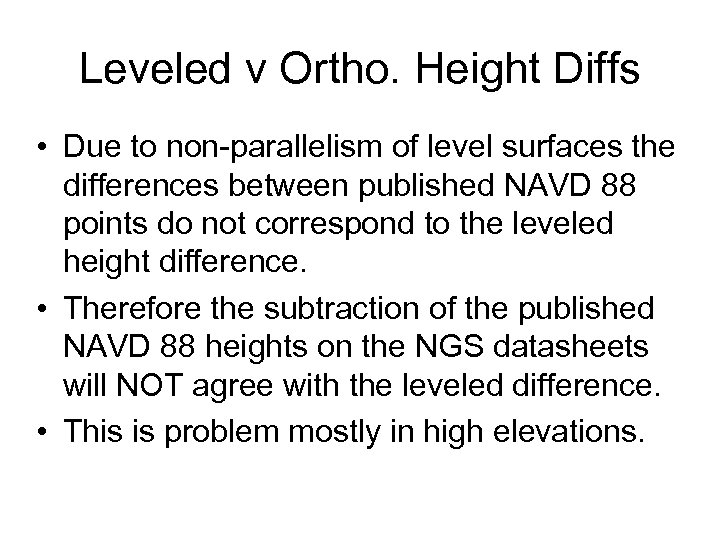Leveled v Ortho. Height Diffs • Due to non-parallelism of level surfaces the differences between published NAVD 88 points do not correspond to the leveled height difference. • Therefore the subtraction of the published NAVD 88 heights on the NGS datasheets will NOT agree with the leveled difference. • This is problem mostly in high elevations.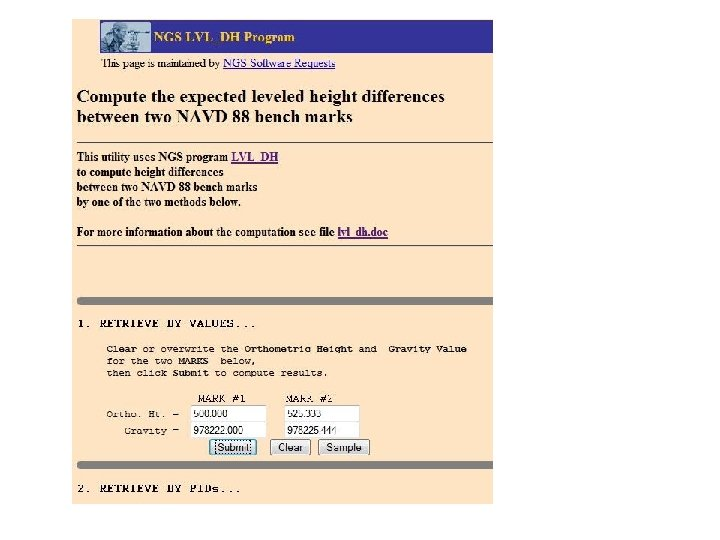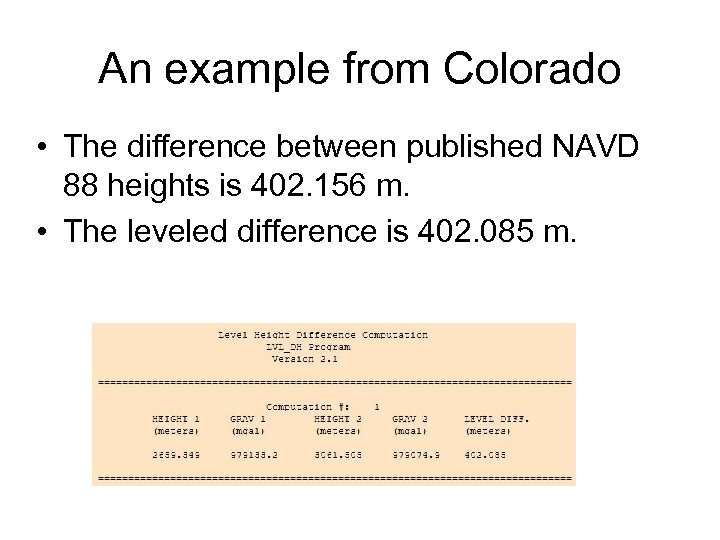An example from Colorado • The difference between published NAVD 88 heights is 402. 156 m. • The leveled difference is 402. 085 m.